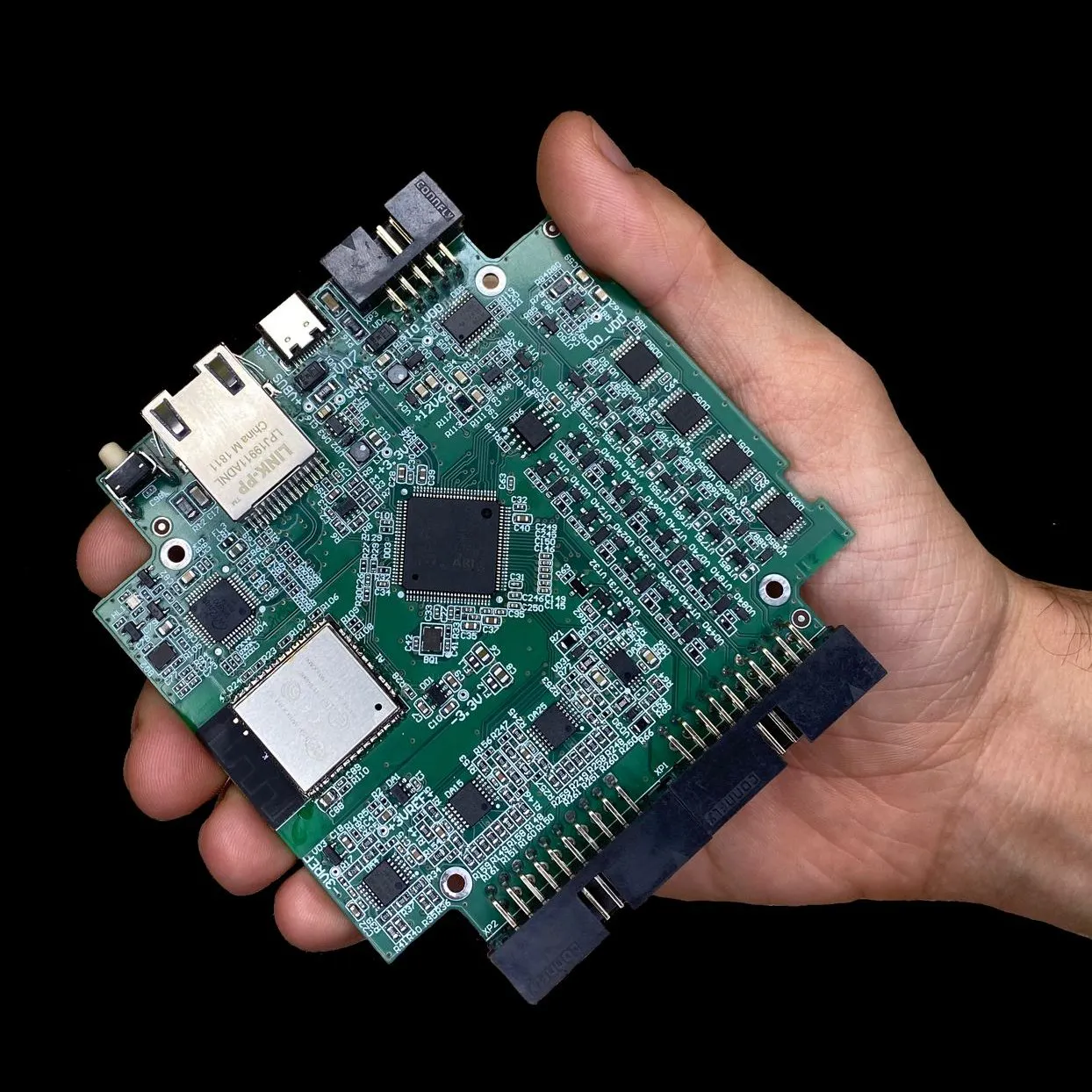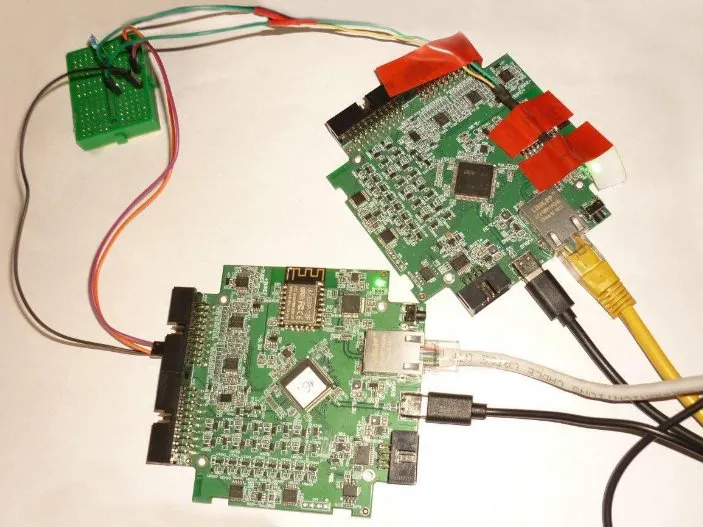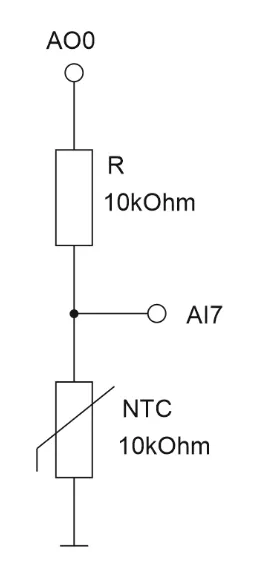Published

# Temperature control of the w5500 chip using a Fork

Quite often in the process of the device development it is necessary to monitor the temperature of individual elements of the circuit

BeginnerFull instructions provided2 hours157FORK
×1

## Schematics

### shema stand## Code

### Python script

Python
```from forkapi.fork import Fork
import math
import matplotlib.pyplot as plt
import matplotlib.animation as animation
import time
import datetime
dev1=Fork('192.168.0.205')

a_res=10000
ntc_res=10000
ntc_b=3950
ao_ch_vcc = 0
ai_ch_ntc1 = 7
ai_ch_ntc2 = 6
max_ch_vcc = 5

def volt_to_res(volt):
return a_res/(max_ch_vcc/volt-1)

def res_to_temp(res):
return 1/( 1/298.15 + 1/ntc_b*math.log(res/ntc_res))

def volt_to_temp(volt):
return res_to_temp(volt_to_res(volt))
dev1.aoSet(ao_ch_vcc, max_ch_vcc)

line1=[]
fig = plt.figure(figsize=(15,8))

_time = []
T1=[]
T2=[]

v = dev1.aiGet(ao_ch_vcc)

v1, v2 = 0,0

# def animate(i, _time, T1,T2,last_v1, last_v2):
def animate(i, _time, T1,T2):

_time.append(datetime.datetime.now())
v1 = dev1.aiGet(ai_ch_ntc1)
t1 = volt_to_temp( max(v1, animate.last_v1)) -273.15
# v1 = max(dev1.aiGet(ai_ch_ntc1), dev1.aiGet(ai_ch_ntc1))
# t1 = volt_to_temp( v1) -273.15
T1.append(t1)
animate.last_v1 = v1

v2 = dev1.aiGet(ai_ch_ntc2)
t2 = volt_to_temp(max(v2, animate.last_v2)) -273.15
# v2 = max(dev1.aiGet(ai_ch_ntc2),dev1.aiGet(ai_ch_ntc2))
# t2 = volt_to_temp(v2) -273.15
T2.append(t2)
animate.last_v2 = v2

print (t1, t2)
plt.cla()
plt.plot(_time, T1)
plt.plot(_time, T2)
plt.ylabel('Temperature, \xB0C')
plt.xticks(rotation=30, ha='right')
labels = [ 'W5500' , 'ambient']
plt.legend(labels, loc = 'best')
animate.last_v1, animate.last_v2 = dev1.aiGet(ai_ch_ntc1),dev1.aiGet(ai_ch_ntc2)
ani = animation.FuncAnimation(fig, animate, fargs=(_time, T1,T2), interval=1000)
plt.show()
```

## Credits

### FORK

11 projects • 1 follower
FORK for test-engineers to automate fixtures. Just take Fork, PyTest and program the tests in Python. The test stand is ready!
Thanks to Fork.